# Trigonometry (Law of Cosines)

• B
Summary:
In case of right angled triangles, C^2 = A^2 +B^2 - 2AB cos(Ψ) is shortened to C^2 = A^2 +B^2 because the cosine of the angle "Ψ" which is 90° is equal to 0. But how is its cosine equal to 0.
I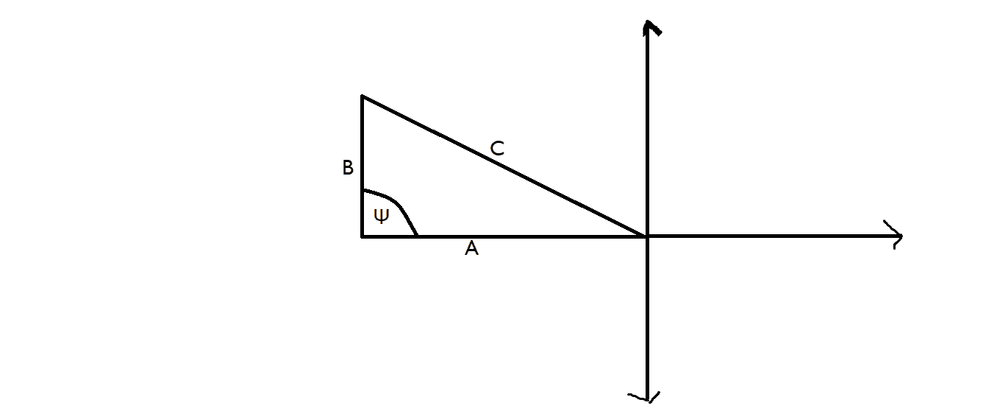etotheipi
##\cos{90^{\circ}} = 0##

•$$\sin^2 \psi + \cos^2 \psi = 1$$
$$if~\psi = 90^{\circ} ~and~accepting ~\sin 90^{\circ} = 1$$

$$1 +\cos^2 90^{\circ} = 1$$
$$\cos^2 90^{\circ} = 0$$
$$\cos 90^{\circ}=0$$

•SSG-E
etotheipi
@Adesh perhaps, but that would rely on knowing ##\sin{90^{\circ}} = 1## and we're back where we started.

There are a few ways of determining the values of trigonometric functions. A useful tool is the so-called unit circle, which consists of a circle of radius 1 centred on the origin in the ##x##-##y## plane. The coordinates of any point on the circle at an angle ##t## to the positive ##x## axis are ##(\cos{t}, \sin{t})##, as shown: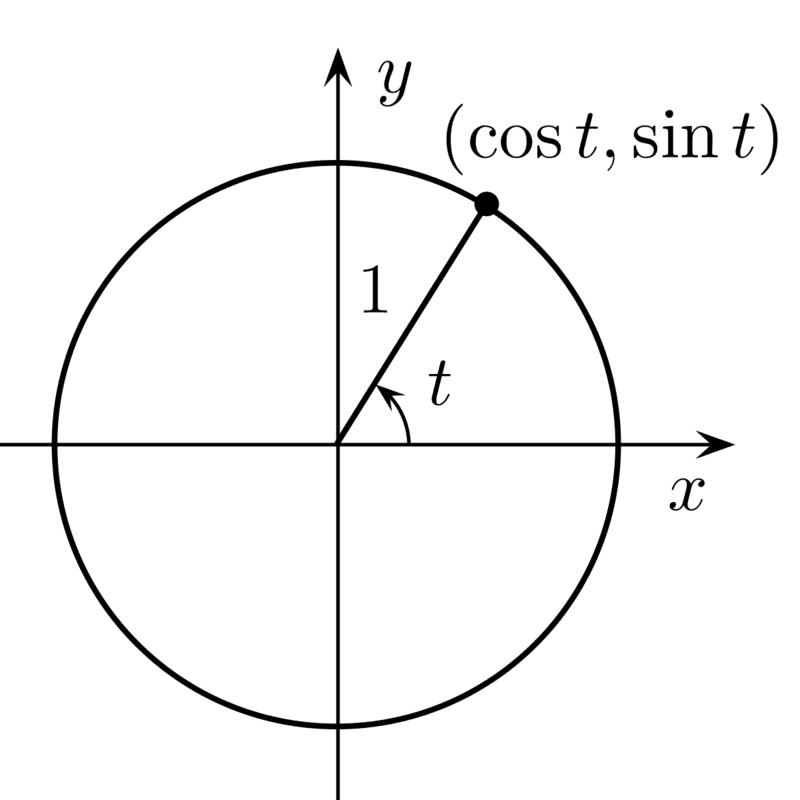If ##t = \frac{\pi}{2} = 90^{\circ}##, the point is at the top of the circle. The ##x##-coordinate is ##0##, and this is the cosine of the angle. It's also useful for determining the signs of trigonometric functions in the different quadrants (I've seen this called the "CAST Diagram" before).

Really you should memorise how ##\sin##, ##\cos## and ##\tan## behave at ##\theta = 0##, ##\theta = \frac{\pi}{6}##, ##\theta = \frac{\pi}{4}##, ##\theta = \frac{\pi}{3}## and ##\theta = \frac{\pi}{2}##. You can use the unit circle and trigonometric identities to deduce the values for lots more angles from them.

•mathwonk, sysprog, Mark44 and 1 other person
symbolipoint
Homework Helper
Gold Member
But how is its cosine equal to 0.
Not Correct.

symbolipoint
Homework Helper
Gold Member
Summary:: In case of right angled triangles, C^2 = A^2 +B^2 - 2AB cos(Ψ) is shortened to C^2 = A^2 +B^2 because the cosine of the angle "Ψ" which is 90° is equal to 0. But how is its cosine equal to 0.

IView attachment 264987
You can find the proof of Law Of Cosines in some Trigonometry books and some Calculus books. There is an old book by Anton which includes a proof.

mathwonk
Homework Helper
2020 Award
My favorite proofs are propositions 12, 13 Book II, Euclid's Elements.

•jim mcnamara
neilparker62
Homework Helper
Summary:: In case of right angled triangles, C^2 = A^2 +B^2 - 2AB cos(Ψ) is shortened to C^2 = A^2 +B^2 because the cosine of the angle "Ψ" which is 90° is equal to 0. But how is its cosine equal to 0.

cos 90 = sin(90-90) = sin(0) = 0.

Does that now beg the question "how is sin(0) = 0" ?

etotheipi
cos 90 = sin(90-90) = sin(0) = 0.

Does that now beg the question "how is sin(0) = 0" ?

But you have turned the question into something that is much easier to answer, if you know or can derive the series expansions. Namely...

##\sin{0} = 0 - \frac{1}{3!}0^3 + \frac{1}{5!}0^5 + \dots = 0##

neilparker62
Homework Helper
Proof of Cosine Rule using Ptolemy's Thm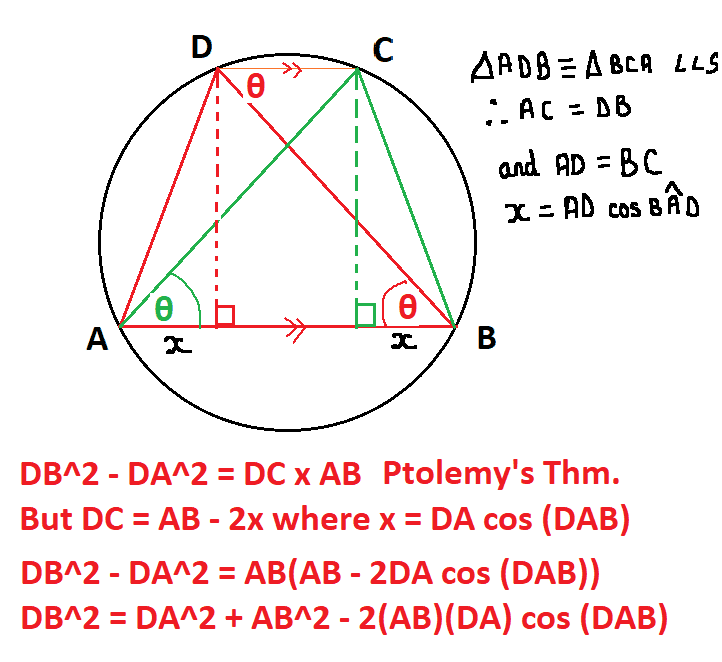Last edited:
neilparker62
Homework Helper
My favorite proofs are propositions 12, 13 Book II, Euclid's Elements.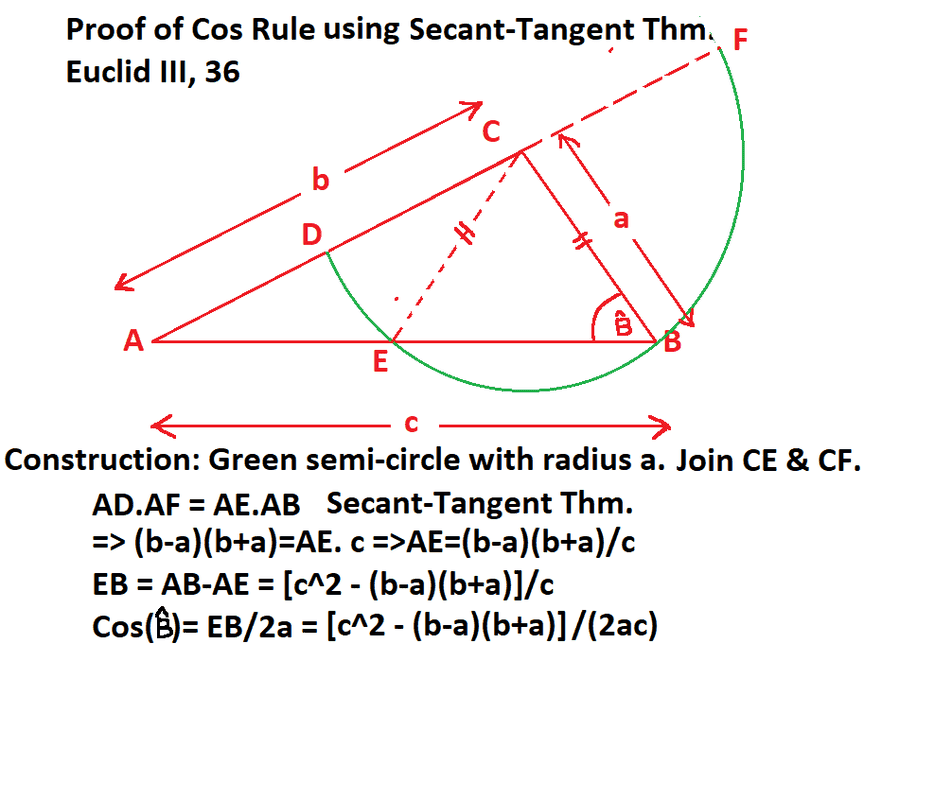etotheipi
Another way, label the triangle like this: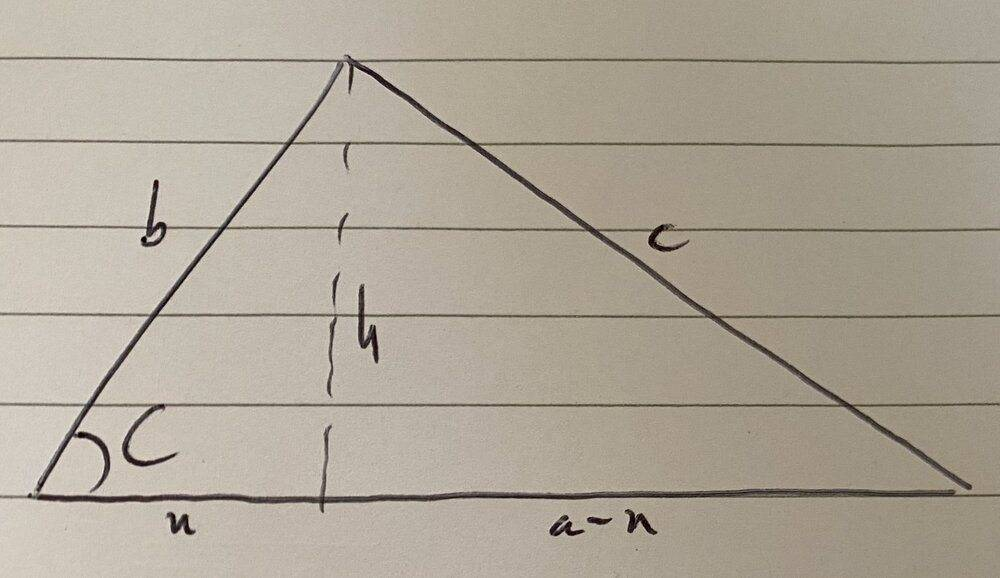Pythagoras on the left triangle: ##x^2 + h^2 = b^2##
Pythagoras on the right triangle: ##a^2 - 2ax + (x^2 + h^2) = c^2##

Now since ##b\cos{C} = x##, we finally have ##a^2 + b^2 - 2ab\cos{C} = c^2##.

•Another way, label the triangle like this:

View attachment 265361

Pythagoras on the left triangle: ##x^2 + h^2 = b^2##
Pythagoras on the right triangle: ##a^2 - 2ax + (x^2 + h^2) = c^2##

Now since ##b\cos{C} = x##, we finally have ##a^2 + b^2 - 2ab\cos{C} = c^2##.Oh you English people! I can see that you have not even used the scale for drawing that perpendicular ##h## and that ##h## is very pointy, it has sharp end points. It’s really an English’s writing (so tough to read).

•etotheipi
etotheipiOh you English people! I can see that you have not even used the scale for drawing that perpendicular ##h## and that ##h## is very pointy, it has sharp end points. It’s really an English’s writing (so tough to read).

Yes my hand-writing is terrible, I never got my pen-license in primary school•Draw an unit circle and add an additional line on the positive y axis as the terminal arm. Let z denote the length of the terminal arm, we get: $$\cos(\theta)=\frac x z\implies \cos(90)=\frac 0 1 = 0$$

Last edited:
etotheipi
Draw an unit circle and add an additional line on the positive y axis as the terminal arm. Let z denote the length of the terminal arm, we get: $$\cos(x)=\frac x z\implies \cos(90)=\frac 0 1 = 0$$

What do you mean by a terminal arm?

Mark44
Mentor
What do you mean by a terminal arm?
I'm pretty sure that he means the ray whose angle is measured relative to the reference direction, the positive x-axis.

•sysprog and (deleted member)
robphy
Homework Helper
Gold Member
In some texts, the law of cosines or a polarization identity is used to define the cosine function:
$$\vec C = \vec A +\vec B$$

\begin{align*} C^2 &= (\vec A+\vec B)\cdot (\vec A+\vec B) =A^2 +B^2 + 2AB\cos\theta \\ \cos\theta_{AB} &\equiv\frac{C^2-A^2-B^2}{2AB} \end{align*}

\begin{align*} (\vec A+\vec B)\cdot (\vec A+\vec B) &=A^2 +B^2 + 2AB\cos\theta \\ (\vec A-\vec B)\cdot (\vec A-\vec B) &=A^2 +B^2 - 2AB\cos\theta \\ \cos\theta_{AB} &\equiv\frac{(\vec A+\vec B)\cdot (\vec A+\vec B) - (\vec A-\vec B)\cdot (\vec A-\vec B)}{4AB} \end{align*}

•etotheipi
neilparker62
Homework Helper
Another way, label the triangle like this: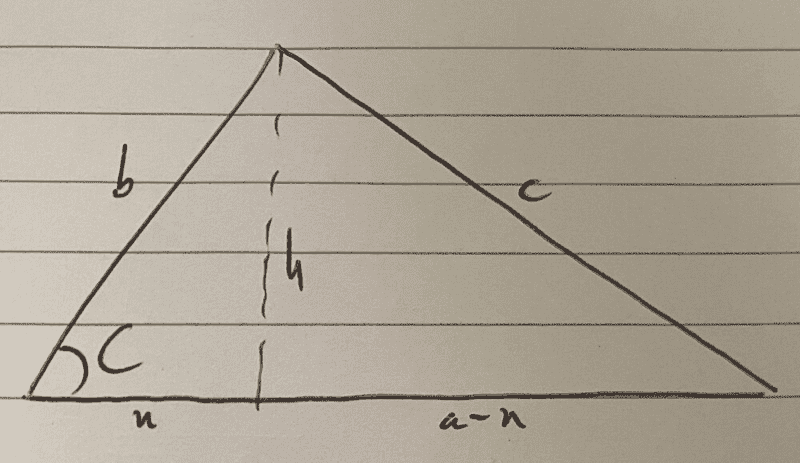Pythagoras on the left triangle: ##x^2 + h^2 = b^2##
Pythagoras on the right triangle: ##a^2 - 2ax + (x^2 + h^2) = c^2##

Now since ##b\cos{C} = x##, we finally have ##a^2 + b^2 - 2ab\cos{C} = c^2##.
##h=b\sin\hat{C}## and ##x=b\cos\hat{C}##. Hence:
$$c^2=b^2\sin^2\hat{C}+(a-b\cos\hat{C})^2=a^2 + b^2 - 2ab\cos{\hat{C}}$$, and as a 'bonus': $$\tan\hat{B}=\frac{b\sin\hat{C}}{a-b\cos\hat{C}}$$

##h=b\sin\hat{C}## and ##x=b\cos\hat{C}##. Hence:
$$c^2=b^2\sin^2\hat{C}+(a-b\cos\hat{C})^2=a^2 + b^2 - 2ab\cos{\hat{C}}$$, and as a 'bonus': $$\tan\hat{B}=\frac{b\sin\hat{C}}{a-b\cos\hat{C}}$$It
It is a great loss of archaeology that you do not work in that field.

•neilparker62
neilparker62
Homework Helper
It is a great loss of archaeology that you do not work in that field.
thanks - cool profile pic by the way. Where is it from ?mathwonk
•